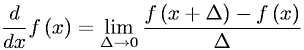Equations > Calculus > Differentiation/Differentals > Definition of a Derivative

### Definition of a DerivativeLatex Code:

MathML Code:

 $\fracd\mathrm{dx}f\left(x\right)=\underset{\Delta \to 0}{lim}\fracf\left(x+\Delta \right)-f\left(x\right)\Delta$

MathType 5.0: